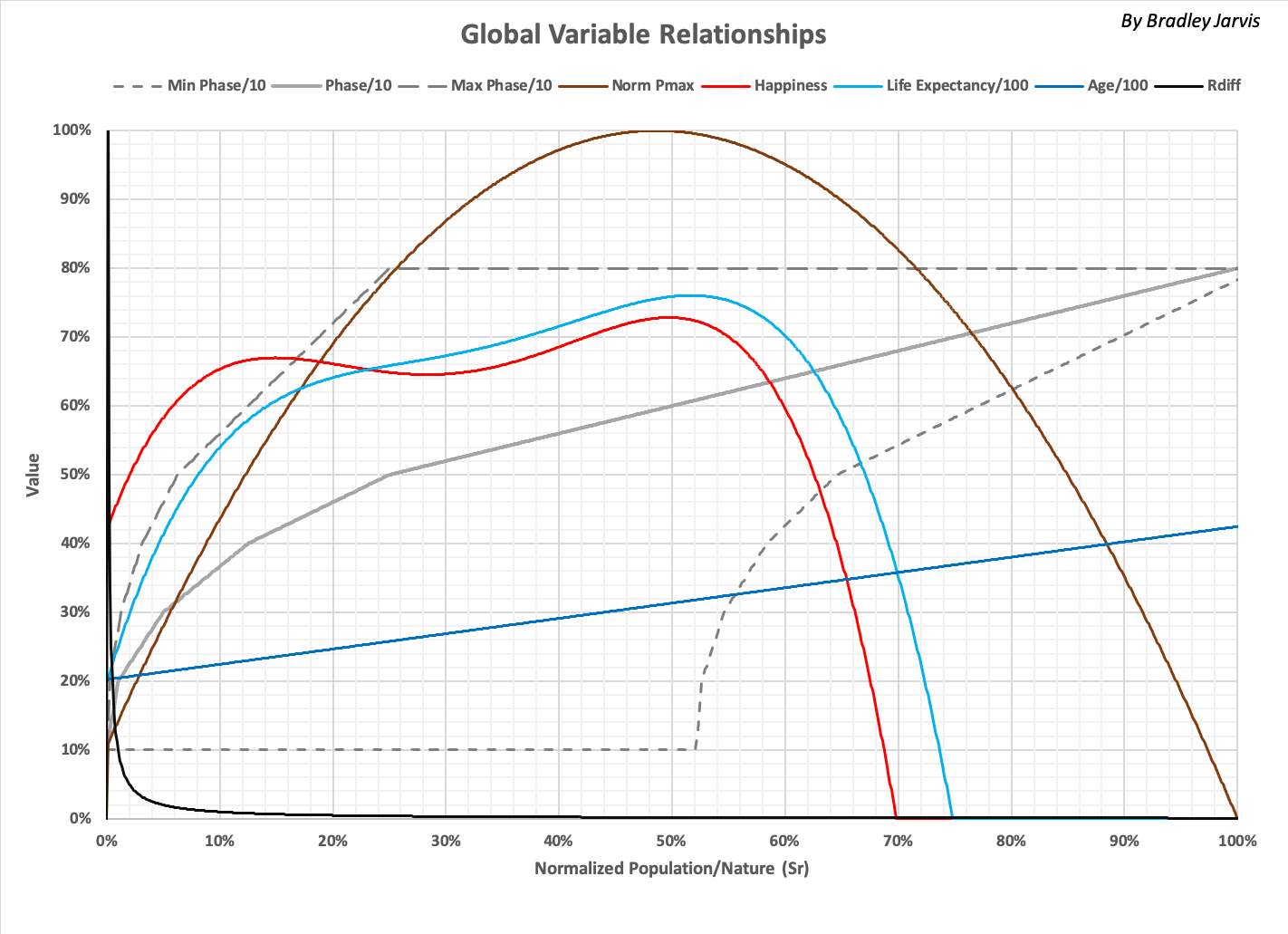# Timelines Model (Version 5)

The following graph shows the relationships between global variables tracked in the Timelines model. Roll over to see the definitions as a function of phase.Definitions of variables and terms used here and elsewhere are as follows. Variable names are capitalized, and references to other entries are italicized.

 adjusted impact Amount of  Impact after it has been changed by human activity (typically Consumption) Age Median age of a population, in years Birth Rate Births per year as a fraction of the population C Average Consumption per person in a group Capacity Total amount of ecological resources available to, and used by, a group (see Rtotal) Cap/p Capacity per person C-low Simulation that reduces consumption at a roughly constant rate to offset external Impact Consumption Ecological footprint of group expressed in ecological resources(see R) cum, cumulative Sum of values for a variable as a function of another variable \$ current U.S. dollars inflation adjusted for a given year Death Rate Deaths per year as a fraction of the Population ecological footprint Measure of ecological products and services used by humanity, typically expressed as a unit of land area ecological resource Unit of ecological footprint corresponding to an average person's annual basic needs Efficiency, E Variable affecting the distribution of Population in a group epoch Period of time corresponding to when part of a group is in a given Phase. global variable A variable that describes a charcteristic of interest for one or more groups Green The simulation that is the best match to historical values of global variables group A set of people with specific Population, Consumption, and Capacity GWP Gross World Product in U.S. dollars (2010 value unless otherwise indicated) GWP/p Average GWP per person in U.S. dollars (2010 value unless otherwise indicated) Happiness, H Life satisfaction of members of a group (on a scale from 0 to 1, typically expressed in %) Impact, self-sustained impact Change in ecological resources (typically a reduction) continually caused by a non-human source Life Expectancy, L Expected lifetime (in years) of a person or group born in a given year Max Phase The maximum phase in a group Min Phase The minimum phase in a group mixed group A group resulting from interaction of groups based on maximum Weights of Population, C, and Capacity Needs Maximum ecological resources consumed for basic survival Normalized Expressed as a fraction of a given value (such as the maximum expected value or value in a given year) Normalized Population / Nature Sratio / 1.126; also: Sr P Cumulative population as a function of a variable such as phase (P = E^Phratio - E). Phase Activity type; each phase is expressed as a fraction of its Sr range Phase Ratio, Phratio Phratio = (Max Phase - Phase) / (Max Phase - Min Phase) Pmax Maximum world population as a function of  Sratio Population The number of people in a group R Total amount of ecological resources consumed by a group (P * C) Rdiff Amount of total Wants consumed by a given group region An arbitrary collection of resources and people (also group) resource Ecological resource Rs Amount of ecological resources remaining in a region or the world, usable by people and other species Rtotal Capacity \$Convert Global inflation of U.S. dollars, as multiple of a value in 2010 simulation Set of mathematical projections of observable variables (typically global variables) that define a universe Sr Normalized Population / Nature Sratio Ratio of minimum Consumption by people (Needs) to remaining resources (P / Rs) state The combined characteristics of an entity (such a group) based on the values of a set of global variables summed group A group with the sums of interacting groups' Population, Consumption, and Capacity \$T Trillion U.S. dollars total consumption The Consumption of everyone in a group universe, world Theoretical reality defined by a simulation, based on history in the real world variable A mathematical quantity whose value is based on the values of other quantities Wants Resources consumed for purposes other than needs (R - Needs) Waste Consumption in excess of half the total resources in the world (R - Rtotal / 2) Wealth Wealth in U.S. dollars (2010 value unless otherwise indicated) Wealth/p Average wealth per person in U.S. dollars (2010 value unless otherwise indicated) Weight Multiplier of a variable used to calculate the value of another variable weighted group A group resulting from groups interacting based on Weights of Population, Consumption, and Capacity world group All the people and regions in the world identified as one group Year Middle of given calendar year (A.C.E.)
The following summary shows the distribution of variables across the world's population for the year 2019, and a time series of values for simulation Green (the best fit to historical data).